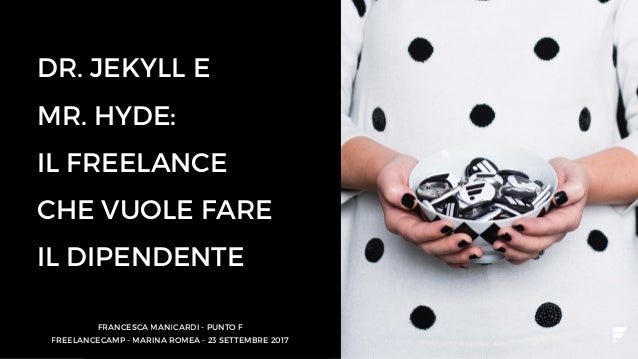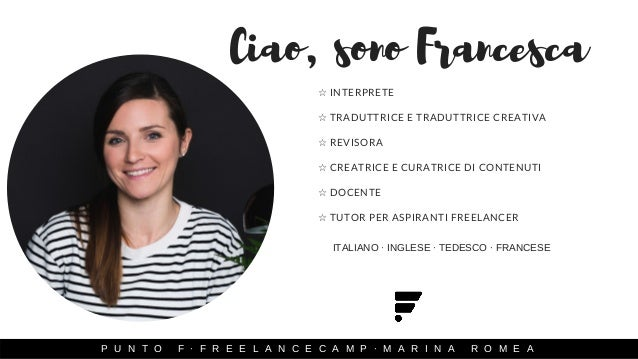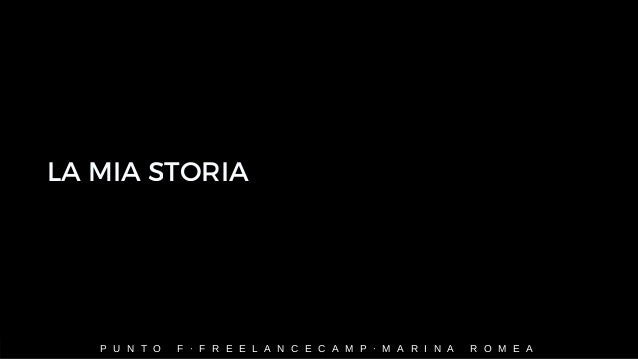Successfully reported this slideshow.You’ve finished this document.
Upcoming SlideShare
Next

ofUpcoming SlideShare
Next

Share

# Dr. Jekyll e Mr. Hyde: il freelance che vuole fare il dipendente

Buone pratiche di convivenza tra lavoro dipendente e lavoro da freelance (se proprio non ne puoi fare a meno).

See all

### Related Audiobooks

#### Free with a 30 day trial from Scribd

See all
• Be the first to like this

### Dr. Jekyll e Mr. Hyde: il freelance che vuole fare il dipendente

1. 1. DR. JEKYLL E MR. HYDE: IL FREELANCE CHE VUOLE FARE IL DIPENDENTE FRANCESCA MANICARDI - PUNTO F FREELANCECAMP - MARINA ROMEA - 23 SETTEMBRE 2017
2. 2. ☆ INTERPRETE   ☆ TRADUTTRICE E TRADUTTRICE CREATIVA ☆ REVISORA ☆ CREATRICE E CURATRICE DI CONTENUTI ☆ DOCENTE ☆ TUTOR PER ASPIRANTI FREELANCER    Ciao, sono Francesca P U N T O F · F R E E L A N C E C A M P · M A R I N A R O M E A ITALIANO · INGLESE · TEDESCO · FRANCESE
3. 3. P U N T O F · F R E E L A N C E C A M P · M A R I N A R O M E A LA MIA STORIA
4. 4. P U N T O F · F R E E L A N C E C A M P · M A R I N A R O M E A Ho aperto partita iva2014 2015 Nasce Punto F Supplenza annuale Supplenza mensile 2015/2016 2017
5. 5. P U N T O F · F R E E L A N C E C A M P · M A R I N A R O M E A PRO
6. 6. P U N T O F · F R E E L A N C E C A M P · M A R I N A R O M E A ☺ MONEY, MONEY, MONEY
7. 7. P U N T O F · F R E E L A N C E C A M P · M A R I N A R O M E A ☺ (FIXED) MONEY, MONEY, MONEY*
8. 8. P U N T O F · F R E E L A N C E C A M P · M A R I N A R O M E A ☺ MONEY, MONEY, MONEY X 13
9. 9. P U N T O F · F R E E L A N C E C A M P · M A R I N A R O M E A ☺ MONEY, MONEY, MONEY - THE END
10. 10. P U N T O F · F R E E L A N C E C A M P · M A R I N A R O M E A ☺ MALATTIA - PORTAMI VIA
11. 11. P U N T O F · F R E E L A N C E C A M P · M A R I N A R O M E A CONTRO
12. 12. P U N T O F · F R E E L A N C E C A M P · M A R I N A R O M E A ☹ NON SEI WONDER WOMAN (O SUPERMAN)
13. 13. P U N T O F · F R E E L A N C E C A M P · M A R I N A R O M E A ☹ VUOI GIOCARE A TETRIS CON ME?
14. 14. P U N T O F · F R E E L A N C E C A M P · M A R I N A R O M E A ☹ LA FORTUNA È CIECA     (MA LA LEGGE DI MURPHY CI VEDE BENISSIMO)
15. 15. P U N T O F · F R E E L A N C E C A M P · M A R I N A R O M E A ☹ ROUTINE vs NON ROUTINE
16. 16. P U N T O F · F R E E L A N C E C A M P · M A R I N A R O M E A ☹ ESSERE O NON ESSERE     (IL CAPO)
17. 17. P U N T O F · F R E E L A N C E C A M P · M A R I N A R O M E A ☹ LET IT GO
18. 18. P U N T O F · F R E E L A N C E C A M P · M A R I N A R O M E A ☹ LE FERIE, CHISSELE*******
19. 19. P U N T O F · F R E E L A N C E C A M P · M A R I N A R O M E A ☹ SOLITUDINE MON AMOUR
20. 20. P U N T O F · F R E E L A N C E C A M P · M A R I N A R O M E A ☹ ALLA RICERCA DELLA MOTIVAZIONE PERDUTA
21. 21. P U N T O F · F R E E L A N C E C A M P · M A R I N A R O M E A ☹ MALATTIA PORTAMI VIA     aka LA (NON) LIBERTÀ DI AMMALARSI
22. 22. P U N T O F · F R E E L A N C E C A M P · M A R I N A R O M E A SPECIAL EDITION
23. 23. P U N T O F · F R E E L A N C E C A M P · M A R I N A R O M E A RAPPORTO CON I GENITORI + COMPITI DA CORREGGERE
24. 24. P U N T O F · F R E E L A N C E C A M P · M A R I N A R O M E A E QUINDI?
25. 25. P U N T O F · F R E E L A N C E C A M P · M A R I N A R O M E A ☞ PENSACI
26. 26. P U N T O F · F R E E L A N C E C A M P · M A R I N A R O M E A ☞ PENSACI ANCORA
27. 27. P U N T O F · F R E E L A N C E C A M P · M A R I N A R O M E A ☞ DATTI UNA SCADENZA
28. 28. P U N T O F · F R E E L A N C E C A M P · M A R I N A R O M E A ☞ NON FARLO!
29. 29. P U N T O F · F R E E L A N C E C A M P · M A R I N A R O M E A "GIURO DI NON FARLO PIÙ, VOSTRO ONORE" cit.
30. 30. P U N T O F · F R E E L A N C E C A M P · M A R I N A R O M E A
31. 31. P U N T O F · F R E E L A N C E C A M P · M A R I N A R O M E A Grazie! WWW.PUNTO-F.COM INFO@PUNTO-F.COM FACEBOOK.COM/PUNTO.F2/ IG @PUNTO_F

Total views

180

On Slideshare

0

From embeds

0

Number of embeds

0

4

Shares

0